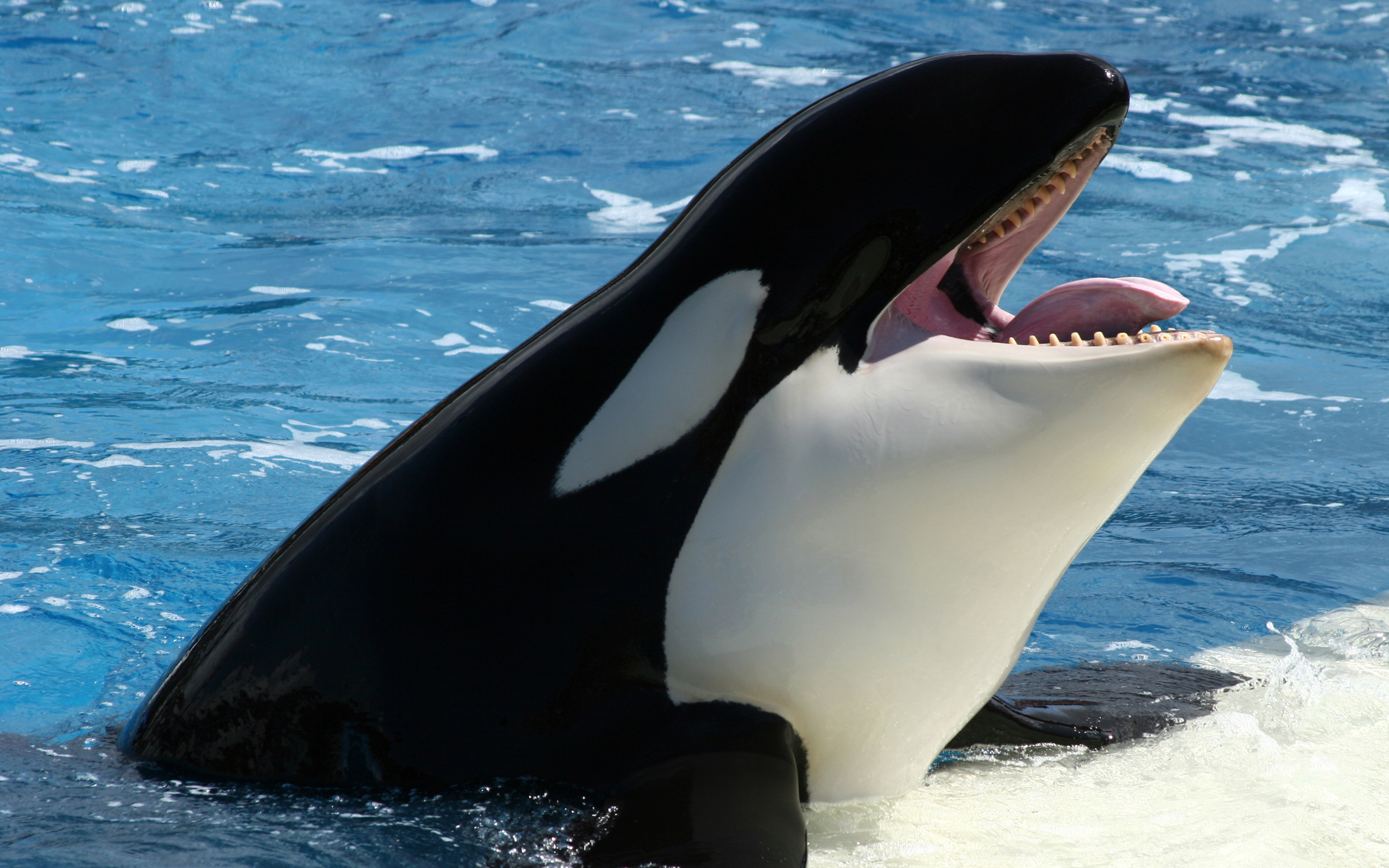# Detection of Orca calls using Active Learning: GSoC progress report #2In this section, we are going to see the steps taken for performing Active learning and how the performance of the model is improved with the help of Active Learning. This blog post would also help us to see how the model labels the sample and how much accuracy on the test data set is improved because of Active Learning.

In the last blog post, we had performed two stages:

1) Preprocessing Stage

2) Model building Stage

This blog post is a continuation of the previous blog post describing the development of the Active Learning pipeline. In the past month, we worked on the Active Learning pipeline, where we would keep aside a subset of data from the training data and train the Basic CNN model (described in the last blog) on the remaining dataset to perform predictions. Depending on the uncertain condition(i.e. if probability prediction of the samples between 0.4 and 0.6: since 1 classifies as no call and 0 classifies as call, the probability prediction closer to 0.5 would mean most uncertain calls) they would either be used by labeled by the experts like Scott and Val or directly be used by the model for retraining. But before starting, please take a look at the preprocessing steps that were taken and the different models that I used in the last blog along with the ROC curve which would help you understand this blog even better.
The steps taken in this diagram are as follows:
1) Preprocess Spectrograms: Generate Melspectrograms with the help of librosa library and then apply PCEN and Wavelet Denoising to these spectrograms.These spectrograms are generated from the audio files containing calls and no calls.
2) Train our CNN model on training data.
Note: A small subset from the training data has been removed for active learning on which the model is not trained on.
3) Test the accuracy of the model on the test data.
4) Use this model to perform probability prediction on the subset of training sample and check if the probability prediction is between 0.4 and 0.6.
5) If yes, ask experts like Scott, Val to label them and pass them to the training directory with True labels.
6) If no, then pass those samples to the training directory with the labels predicted by the model.
7) Retrain the model with this new data along with the old data.
8) Check the accuracy of the model on test data.

Fig. 1. Active Learning pipeline

Here,is the distribution of the training data, active-learning data, and test data.

Stage 1: Preprocess Spectrograms: Here I am going to apply PCEN and Wavelet-Denoising on the spectrograms extracted from the audio data.

(This part is explained in detail in Blog 1)

Stage 2: We are going to train our CNN model on the 1394 training samples and determine the accuracy of the model on the test dataset.

The accuracy of the test dataset before active learning is:

[[80 21]
[14 86]]
acc: 0.8259
sensitivity: 0.7921
specificity: 0.8600

Stage 3: We are going to use this trained model and perform prediction on the other sample which consists of 176 samples.

Stage 4: In this stage, we are going to pass the uncertain samples to the user for labeling. The model predicts that 0 for calls and 1 for no calls so if the probability predicted by the model is closer to 0 we would identify it as call and vice-versa. A model would be uncertain when the probability prediction of the particular sample is closer to 0.5.

The predicted calls with a  probability prediction between the values 0.4 to 0.6 were selected to be passed to the expert for labeling.

The expert labeled  samples are  then passed to the training directory. Here out of 176 samples, the model had 36 uncertain samples.

The other samples that the model classifies slightly more confidently than these are directly moved to the training folder without asking the expert. the model predicts a  sample with a probability prediction less than 0.4, then it is directly passed to the call directory of the training dataset. If the model predicts the sample with probability prediction greater than 0.6, then the samples are  passed to the no calls folder of the training directory

Stage 5: Start retraining of the model, on these newly placed samples with the older ones. Therefore the total number of samples containing both calls and no calls combined would be 1394 (the old ones on which the model was trained on) plus the new 176 samples (that the model classified and placed into respective calls and no calls folders with the uncertain calls correctly classified by the expert).

Therefore, after retraining it on 1570 images, we would perform prediction on the test dataset to see the improvement of the active learning phase.

Here are the results:

Found 201 images belonging to 2 classes.

[[78 23]
[10 90]]
acc: 0.8358
sensitivity: 0.7723
specificity: 0.9000

We find that Active Learning improved the results by approximately  1%. I hope you found this blog useful and thank you for your time and efforts in reading this blog!